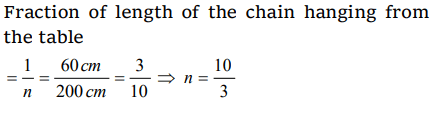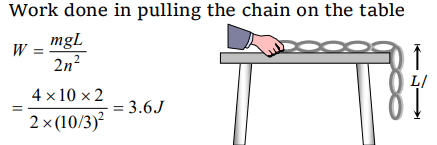## Work, Energy and Power Questions and Answers Part-3

1. A 50kg man with 20kg load on his head climbs up 20 steps of 0.25m height each. The work done in climbing is
a) 5 J
b) 350 J
c) 100 J
d) 3430 J

Explanation: Total mass = (50 + 20) = 70 kg
Total height = 20 × 0.25 = 5m
Work done = mgh = 70 × 9.8 × 5 = 3430 J

2. A force $\vec{F}=6\hat{i}+2\hat{j}-3\hat{k}$    acts on a particle and produces a displacement of $\vec{s}=2\hat{i}-3\hat{j}+x\hat{k}$    . If the work done is zero, the value of x is
a) – 2
b) $1\diagup2$
c) 6
d) 2

Explanation: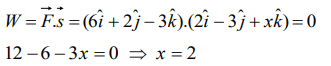3. A force $\left(\vec{F}\right)=3\hat{i}+c\hat{j}+2\hat{k}$    acting on a particle causes a displacement: $\left(\vec{s}\right)=-4\hat{i}+2\hat{j}+3\hat{k}$    in its own direction. If the work done is , 6 J then the value of 'c' is
a) 0
b) 1
c) 6
d) 12

Explanation: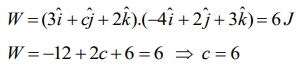4. In an explosion a body breaks up into two pieces of unequal masses. In this
a) Both parts will have numerically equal momentum
b) Lighter part will have more momentum
c) Heavier part will have more momentum
d) Both parts will have equal kinetic energy

Explanation: Both part will have numerically equal momentum and lighter part will have more velocity

5. Which of the following is a unit of energy
a) Unit
b) Watt
c) Horse Power
d) None

Explanation: Watt and Horsepower are the unit of power

6. If force and displacement of particle in direction of force are doubled. Work would be
a) Double
b) 4 times
c) Half
d) $\frac{1}{4}times$

Explanation: Work = Force × Displacement
If force and displacement both are doubled then work would be four times

7.A body of mass 5 kg is placed at the origin, and can move only on the x-axis. A force of 10 N is acting on it in a direction making an angle of $60^{\circ}$ with the x-axis and displaces it along the x-axis by 4 metres. The work done by the force is
a) 2.5 J
b) 7.25 J
c) 40 J
d) 20 J

Explanation: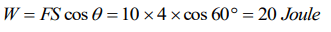8. A force $\vec{F}=\left(5\hat{i}+4\hat{j}\right)$  N acts on a body and produces a displacement $\vec{S}=\left(6\hat{i}-5\hat{j}+3\hat{k}\right)$  m. The work done will be
a) 10 J
b) 20 J
c) 30 J
d) 40 J

Explanation:9. A uniform chain of length 2m is kept on a table such that a length of 60cm hangs freely from the edge of the table. The total mass of the chain is 4kg. What is the work done in pulling the entire chain on the table
a) 7.2 J
b) 3.6 J
c) 120 J
d) 1200 J